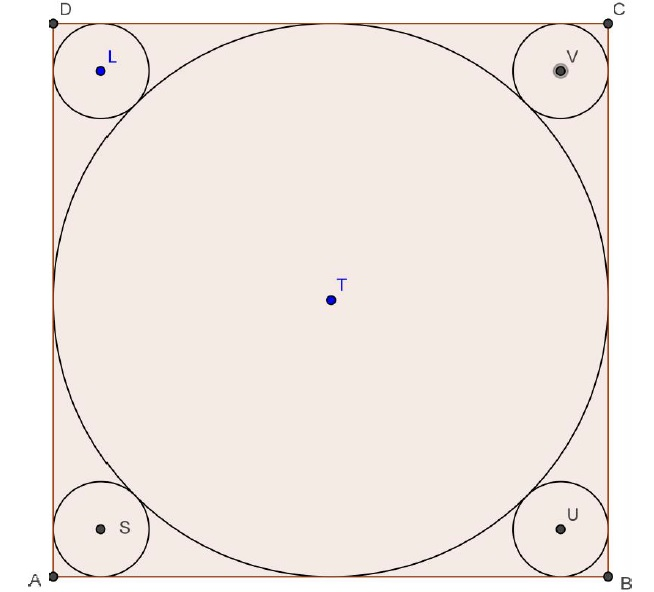# 4 Small Circles

Geometry Level 2ABCD is a square.

Circle T is inscribed in the square and has a radius R.

The small circles are tangent to the circle T and have radius r.

The fraction r/R can be written as a+b√c.

How much is a+b+c ?

×